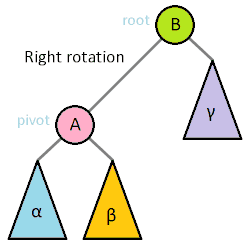# AVL Trees

• Recall that balanced BSTs maintain $h = O(\log n)$, so all operations run in $O(\log n)$ time.

• Self-balancing BSTs are data types that automatically keep track of their height to maintain the balanced property.

• One of the most important operations on most self-balancing binary trees is the rotation. Left and right rotations are animated below.• Some of the popular data structures that implement self-balancing BSTs include:

• 2-3 trees

• AVL trees

• Red-black trees

In this section, we’ll discuss AVL trees.

• AVL trees are the first example (invented in 1962) of a self-balancing binary search tree.

• AVL trees satisfy the height-balance property: for any node $n$, the heights of $n$’s left and right subtrees can differ by at most 1.

• To make math easier, we can define each null node to have height of -1. This will make balancing easier.

• As part of data structure augmentation, each node stores its height. Alternatively, you can store just difference in heights.

• We will call a node unbalanced if its left and right children differ in height by more than 1.

## AVL Find

• The find operation for an AVL tree exactly the same as for a binary search tree.

• Since an AVL tree is guaranteed to be balanced, find always takes $\Theta(\log n)$ time in the worst case, where $n$ is the number of elements in the AVL tree.

## AVL Insert

• To insert into an AVL tree,

1. Insert as into a simple BST.

2. Work your way up the tree, restoring the AVL property and updating heights as you go.

• Take a look at the slides for an example of inserting a sequence of numbers.

## AVL Remove

• Removing a node from an AVL tree falls into one of the three cases:

1. The node is a leaf. This is easy. Simply delete the node.

2. The node has just one child. This is just as easy. Simply delete the node and connect the parent of the deleted node to the child of the deleted node.

3. The node has two children. This is a bit more complicated.

We define the in-order predecessor of a node as the previous node in the in-order traversal of the tree. To find the in-order predecessor, go to left subtree and find the right-most node.

We define the in-order successor of a node as the next node in the in-order traversal of a tree. To find the in-order successor, go to right subtree and find the left-most node.

In order to delete a node that has two children, replace it with either its in-order predecessor or in-order successor.

• After deleting from an AVL, you might need to re-balance the tree.

## AVL Tree Performance

• For an AVL tree with $N$ nodes, searching, insertion and deletion each take $O(\log N)$ time.

• The path from the root to the deepest leaf in an AVL tree is at most $\sim1.44 \log(N + 2)$.

• In addition, AVL trees have high constant factors for some operations. For example, restructuring is an expensive operation, and an AVL tree may have to re-balance itself $\log_2 n$ in the worst case during a removal of a node.